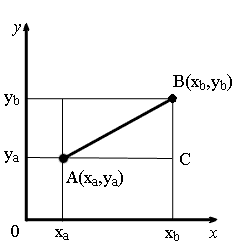Study of mathematics online.
Study math with us and make sure that "Mathematics is easy!"

# Distance between two points

Definition. Distance between two points is the length of the line segment that connects this points.

## Distance formulas:

• The formula for calculating the distance between two points A(xaya) and B(xbyb) on plane:
AB = √(xb - xa)2 + (yb - ya)2
• The formula for calculating the distance between two points A(xayaza) and B(xbybzb) in space:
AB = √(xb - xa)2 + (yb - ya)2 + (zb - za)2

## Proof of the formula of distance between two points for the plane problemFrom the points A and B drop perpendiculars to the coordinate axes.

Consider a right triangle ∆ABC. Legs of the triangle are equal to:

AC = xb - xa;
BC = yb - ya.

Using the Pythagorean theorem, calculate the length of the hypotenuse AB:

AB = √AC2 + BC2.

Substituting to this expression the lengths of AC and BC which expressed in terms of the coordinates of points A and B, we obtain the formula for calculating the distance between points on the plane.

Proof of the formula for calculating the distance between two points in space is similar.

## Examples of tasks with distance between two points

### Examples of tasks with distance between two points on 2D

Example 1.
Find the distance between two points A(-1, 3) and B(6,2).

Solution.

AB = √(xb - xa)2 + (yb - ya)2 = √(6 - (-1))2 + (2 - 3)2 = √72 + 12 = √50 = 5√2

Example 2.
Find the distance between two points A(0, 1) and B(2,-2).

Solution.

AB = √(xb - xa)2 + (yb - ya)2 = (2 - 0)2 + (-2 - 1)2 = 22 + (-3)2 = √13

### Examples of tasks with distance between two points on 3D

Example 3.
Find the distance between two points A(-1, 3, 3) and B(6, 2, -2).

Solution.

AB = √(xb - xa)2 + (yb - ya)2 + (zb - za)2 =

= √(6 - (-1))2 + (2 - 3)2 + (-2 - 3)2 = √72 + 12 + 52 = √75 = 5√3

Example 4.
Find the distance between two points A(0, -3, 3) and B(3, 1, 3).

Solution.

AB = √(xb - xa)2 + (yb - ya)2 + (zb - za)2 =

= √(3 - 0)2 + (1 - (-3))2 + (3 - 3)2 = 32 + 42 + 02 = √25 = 5